# js逆向安全指南（3）-- webpack解包指南

## 逆向目标

• 主页：aHR0cHM6Ly93d3cuZ205OS5jb20v
• 接口：aHR0cHM6Ly9wYXNzcG9ydC5nbTk5LmNvbS9sb2dpbi9sb2dpbjM=
• 逆向参数：Query String Parameters：
password: kRtqfg41ogc8btwGlEw6nWLg8cHcCW6R8JaeM......

## 逆向过程

### 抓包分析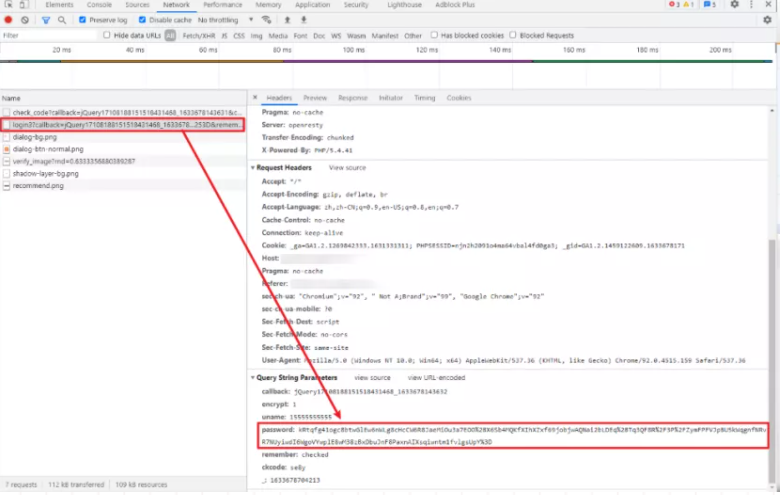### 加密入口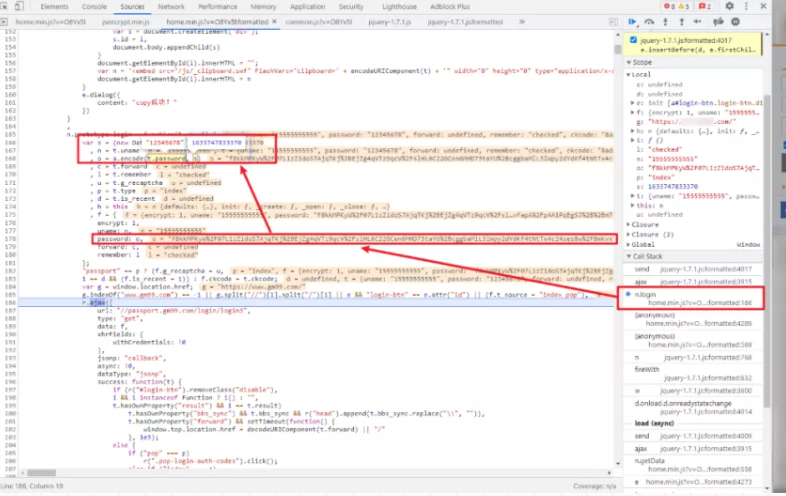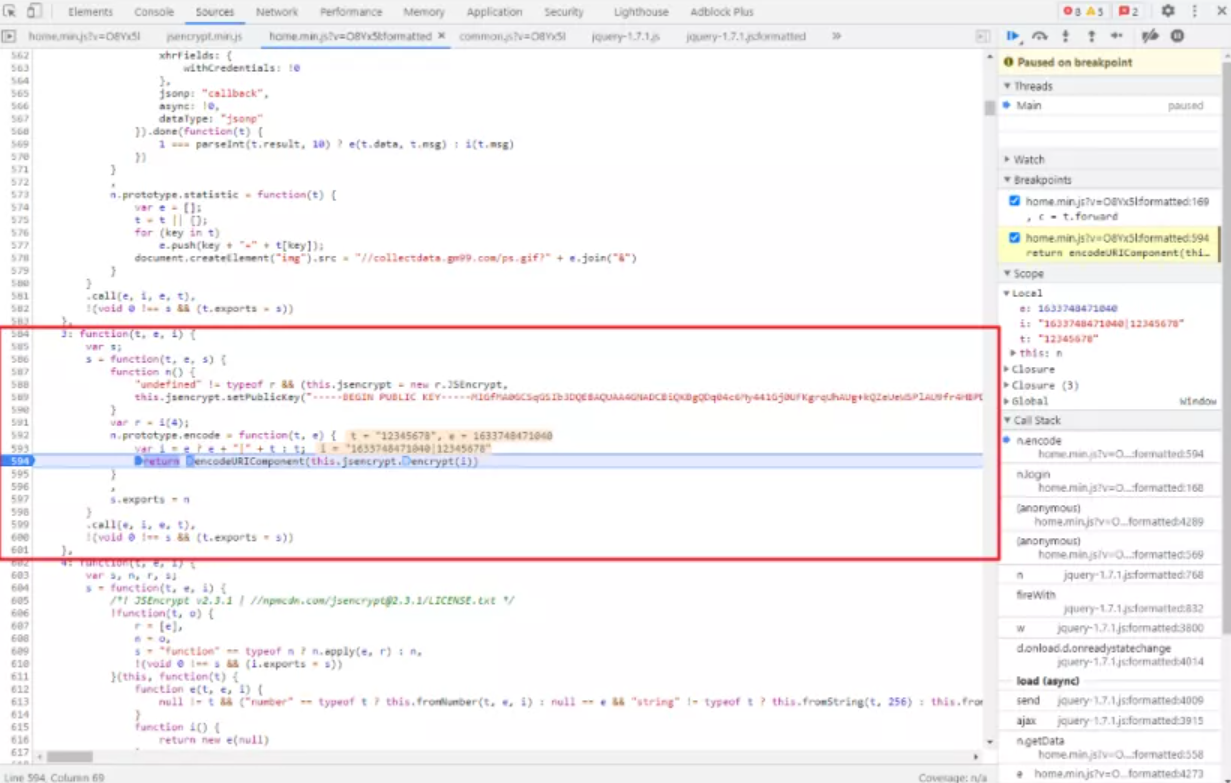RSA 加密找到了公钥，其实就可以直接使用 Python 的 Cryptodome 模块来实现加密过程了，代码如下所示：

import time
import base64
from urllib import parse
from Cryptodome.PublicKey import RSA
from Cryptodome.Cipher import PKCS1_v1_5

timestamp = str(int(time.time() * 1000))
encrypted_object = timestamp + "|" + password
rsa_key = RSA.import_key(base64.b64decode(public_key))  # 导入读取到的公钥
cipher = PKCS1_v1_5.new(rsa_key)                        # 生成对象


/*

*/

JSEncrypt = require("jsencrypt")

var jsEncrypt = new JSEncrypt();
var i = e ? e + "|" + t : t;
return encodeURIComponent(jsEncrypt.encrypt(i));
}

var timestamp = (new Date).getTime();


### webpack 改写

!function (allModule) {
function useModule(whichModule) {
allModule[whichModule].call(null, "hello world!");
}
}([
function module0(param) {console.log("module0: " + param)},
function module1(param) {console.log("module1: " + param)},
function module2(param) {console.log("module2: " + param)},
]);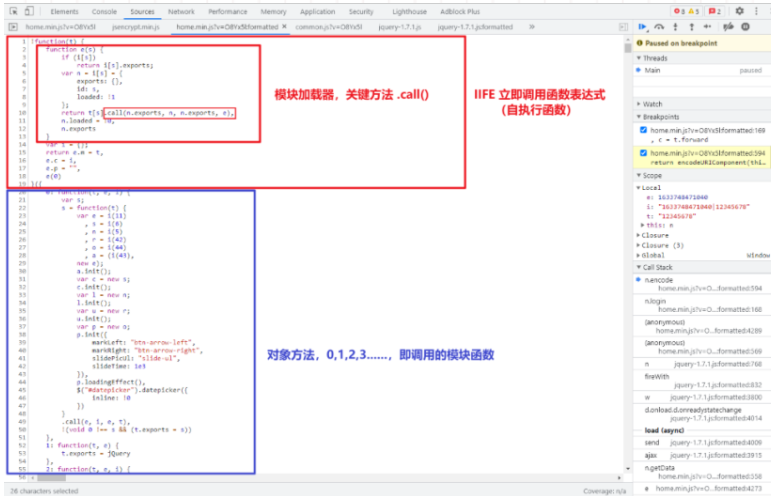#### 1、找到 IIFE

IIFE 立即调用函数表达式，也称为立即执行函数，自执行函数，将源码中的 IIFE 框架抠出来，后续将有用的代码再往里面放：

!function (t) {

}({

})


#### 2、找到模块加载器

!function (t) {
function e(s) {
if (i[s])
return i[s].exports;
var n = i[s] = {
exports: {},
id: s,
};
return t[s].call(n.exports, n, n.exports, e),
n.exports
}
var i = {};
}({

})


#### 3、找到调用的模块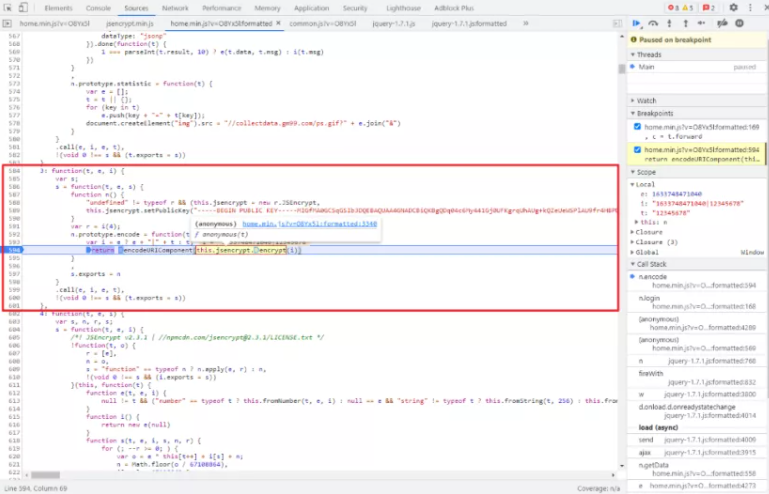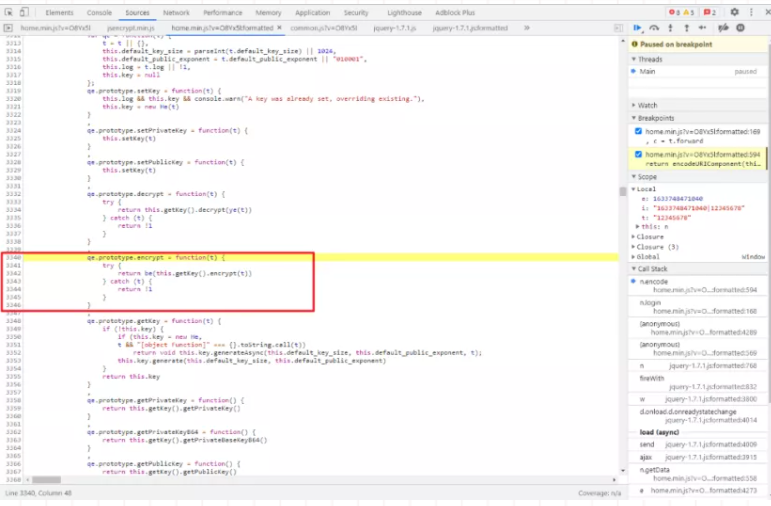!function (t) {
function e(s) {
if (i[s])
return i[s].exports;
var n = i[s] = {
exports: {},
id: s,
};
return t[s].call(n.exports, n, n.exports, e),
n.exports
}
var i = {};
}(
{
4: function (t, e, i) {},
3: function (t, e, i) {
var s;
s = function (t, e, s) {
function n() {
"undefined" != typeof r && (this.jsencrypt = new r.JSEncrypt,
this.jsencrypt.setPublicKey("-----BEGIN PUBLIC KEY-----略-----END PUBLIC KEY-----"))
}

var r = i(4);
n.prototype.encode = function (t, e) {
var i = e ? e + "|" + t : t;
return encodeURIComponent(this.jsencrypt.encrypt(i))
},
s.exports = n
}.call(e, i, e, t),
!(void 0 !== s && (t.exports = s))
}
}
)


#### 4、导出加密函数

var eFunc;

!function (t) {
function e(s) {
if (i[s])
return i[s].exports;
var n = i[s] = {
exports: {},
id: s,
};
return t[s].call(n.exports, n, n.exports, e),
n.exports
}
var i = {};
eFunc = e
}(
{
4: function (t, e, i) {},
3: function (t, e, i) {}
}
)


function getEncryptedPassword(password) {
var timestamp = (new Date).getTime();
var encryptFunc = eFunc("3");
var encrypt = new encryptFunc;
}


var navigator = {};
var window = global;


## 完整代码

GitHub 关注 K 哥爬虫，持续分享爬虫相关代码！欢迎 star ！https://github.com/kgepachong/

### JavaScript 加密关键代码架构

var navigator = {};
var window = global;
var eFunc;

!function (t) {
function e(s) {
if (i[s])
return i[s].exports;
var n = i[s] = {
exports: {},
id: s,
};
return t[s].call(n.exports, n, n.exports, e),
n.exports
}

var i = {};
eFunc = e;
}(
{
4: function (t, e, i) {},
3: function (t, e, i) {}
}
)

var timestamp = (new Date).getTime();
var encryptFunc = eFunc("3");
var encrypt = new encryptFunc;
}

// 测试样例


/*

1、使用 require 语句引用，前提是使用 npm 安装过；
2、将 jsencrypt.js 直接粘贴到此脚本中使用，同时要将结尾 exports.JSEncrypt = JSEncrypt; 改为 je = JSEncrypt 导出方法。
PS：需要定义 var navigator = {}; var window = global;，否则提示未定义。
*/

// ========================= 1、require 方式引用 =========================
// var je = require("jsencrypt")

// =================== 2、直接将 jsencrypt.js 复制过来 ===================
/*! JSEncrypt v2.3.1 | https://npmcdn.com/jsencrypt@2.3.1/LICENSE.txt */
var navigator = {};
var window = global;

// 这里是 jsencrypt.js 代码

var jsEncrypt = new je();
var e = (new Date).getTime();
var i = e ? e + "|" + t : t;
return encodeURIComponent(jsEncrypt.encrypt(i));
}

// 测试样例


### Python 登录关键代码

#!/usr/bin/env python3
# -*- coding: utf-8 -*-

import re
import json
import time
import random
import base64
from urllib import parse

import execjs
import requests
from PIL import Image
from Cryptodome.PublicKey import RSA
from Cryptodome.Cipher import PKCS1_v1_5

verify_image_url = '脱敏处理，完整代码关注 GitHub：https://github.com/kgepachong/crawler'
check_code_url = '脱敏处理，完整代码关注 GitHub：https://github.com/kgepachong/crawler'

'User-Agent': 'Mozilla/5.0 (Windows NT 10.0; Win64; x64) AppleWebKit/537.36 (KHTML, like Gecko) Chrome/91.0.4472.124 Safari/537.36'
}
session = requests.session()

def get_jquery():
jsonp = ''
for _ in range(21):
jsonp += str(random.randint(0, 9))
jquery = 'jQuery' + jsonp + '_'
return jquery

def get_dict_from_jquery(text):
result = re.findall(r'$$(.*?)$$', text)

# 两个 JavaScript 脚本，两种方法均可
with open('gm99_encrypt.js', 'r', encoding='utf-8') as f:
# with open('gm99_encrypt_2.js', 'r', encoding='utf-8') as f:

timestamp = str(int(time.time() * 1000))
encrypted_object = timestamp + "|" + password
public_key = "脱敏处理，完整代码关注 GitHub：https://github.com/kgepachong/crawler"
rsa_key = RSA.import_key(base64.b64decode(public_key))  # 导入读取到的公钥
cipher = PKCS1_v1_5.new(rsa_key)                        # 生成对象

def get_verify_code():
with open('code.png', 'wb') as f:
f.write(response.content)
image = Image.open('code.png')
image.show()
code = input('请输入图片验证码: ')
return code

def check_code(code):
timestamp = str(int(time.time() * 1000))
params = {
'callback': get_jquery() + timestamp,
'ckcode': code,
'_': timestamp,
}
result = get_dict_from_jquery(response.text)
if result['result'] == 1:
pass
else:
raise Exception('验证码输入错误！')

timestamp = str(int(time.time() * 1000))
params = {
'callback': get_jquery() + timestamp,
'encrypt': 1,
'remember': 'checked',
'ckcode': code,
'_': timestamp
}
result = get_dict_from_jquery(response.text)
print(result)

def main():
# 测试账号：15434947408，密码：iXqC@aJt8fi@VwV

# 获取加密后的密码，使用 Python 或者 JavaScript 实现均可

# 获取验证码
code = get_verify_code()

# 校验验证码
check_code(code)

# 登录

if __name__ == '__main__':
main()


## 参考资料

JSEncrypt GitHub: https://github.com/travist/jsencrypt

------------------------------------------------------------------------------------------------------------------------------------------------------------------

### 总结下，webpack解包导出流程：

#### 5、调用加密函数，得出值

posted @ 2021-11-16 18:03  Eeyhan  阅读(1477)  评论(0编辑  收藏  举报